# 🔥【建议收藏】7个面试必考的正则表达式，我不想再遇到了## 1.# 数字价格千分位分割

`这道题估计大家在面试和工作中也经常遇到，出现频率比较高。`

### 正则结果

``````'123456789'.replace(/(?!^)(?=(\d{3})+\$)/g, ',') // 123,456,789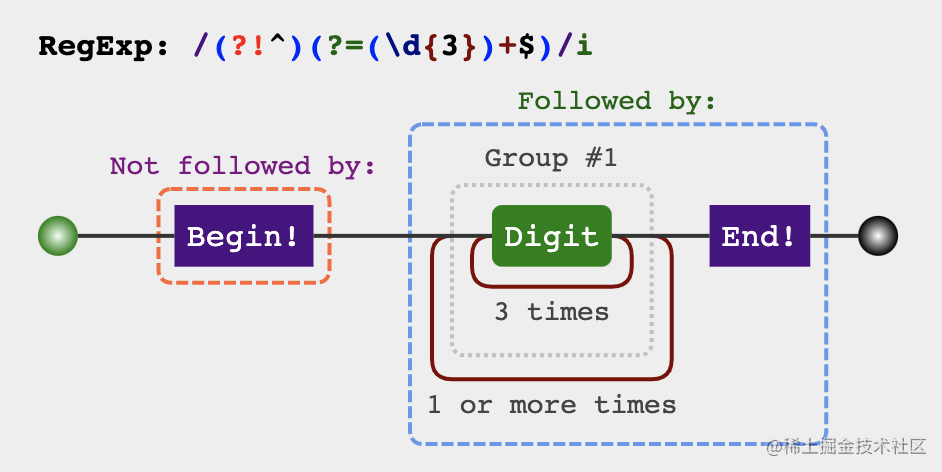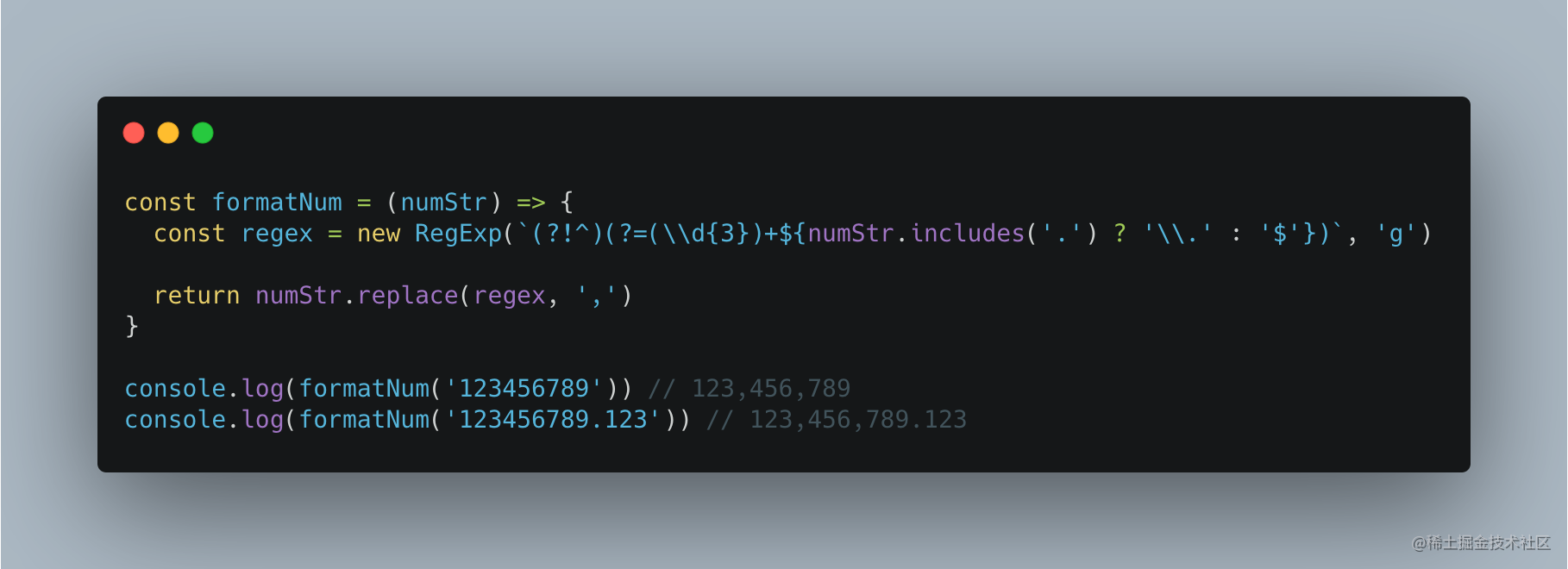### 分析过程

1. 从后往前`每三个数字`前加一个逗号

2. 开头不能加逗号(比如：`123` 最后不能变成`,123`)

``````
let price = '123456789'
let priceReg = /(?=\d{3}\$)/

console.log(price.replace(proceReg, ',')) // 123456,789

``````
let price = '123456789'
let priceReg = /(?=(\d{3})+\$)/g

console.log(price.replace(priceReg, ',')) // ,123,456,789

``````
let price = '123456789'
let priceReg = /(?!^)(?=(\d{3})+\$)/g

console.log(price.replace(priceReg, ',')) // 123,456,789

## 2.# 手机号3-4-4分割

`表单搜集场景，经常遇到的手机格式化`

### 正则结果

``````let mobile = '18379836654'
let mobileReg = /(?=(\d{4})+\$)/g

console.log(mobile.replace(mobileReg, '-')) // 183-7983-6654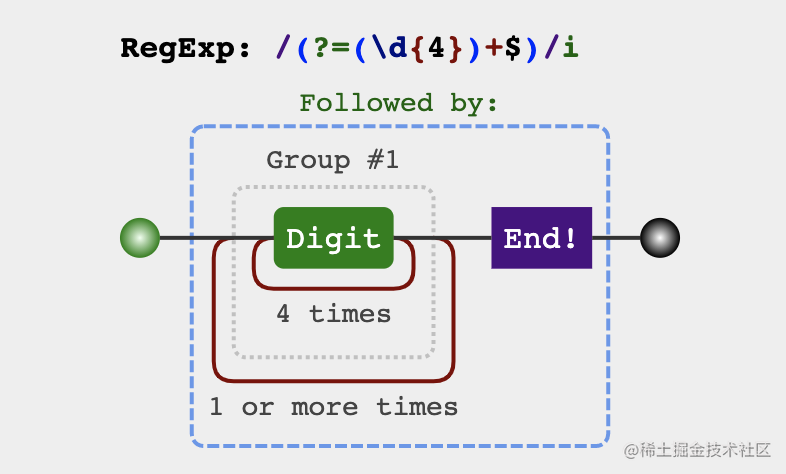### 分析过程

``````
let mobile = '18379836654'
let mobileReg = /(?=(\d{4})+\$)/g

console.log(mobile.replace(mobileReg, '-')) // 183-7983-6654

## 3.# 将字符串驼峰化

``````1. foo Bar => fooBar

2. foo-bar---- => fooBar

3. foo_bar__ => fooBar

### 正则结果

``````const camelCase = (string) => {
const camelCaseRegex = /[-_\s]+(.)?/g

return string.replace(camelCaseRegex, (match, char) => {
return char ? char.toUpperCase() : ''
})
}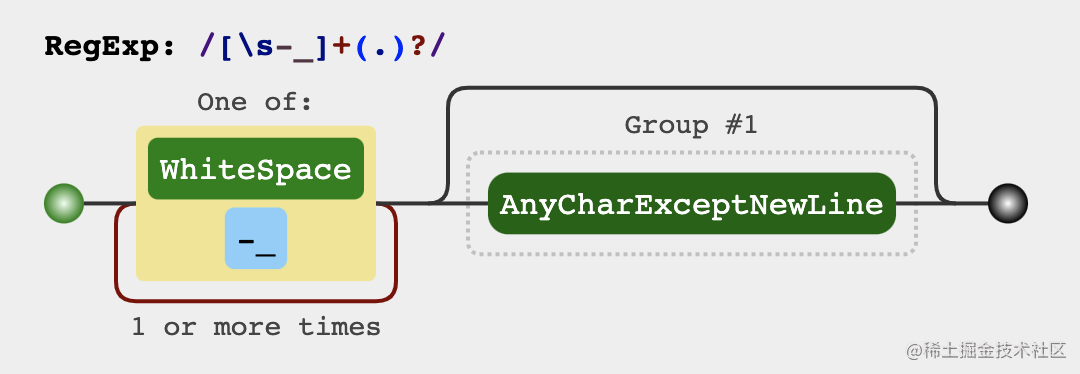### 分析过程

1. 每个单词的前面都有0个或者多个`-` `空格` `_` 如(`Foo``--foo``__FOO``_BAR`` Bar`)
2. `-` `空格` `_`后面有可能不跟任何东西 如(`__``--`)
``````const camelCase = (string) => {
// 注意(.)?这里的?是为了满足条件2
const camelCaseRegex = /[-_\s]+(.)?/g

return string.replace(camelCaseRegex, (match, char) => {
return char ? char.toUpperCase() : ''
})
}

console.log(camelCase('foo Bar')) // fooBar
console.log(camelCase('foo-bar--')) // fooBar
console.log(camelCase('foo_bar__')) // fooBar

## 4.# 将字符串首字母转化为大写，剩下为小写

### 正则结果

``````
const capitalize = (string) => {
const capitalizeRegex = /(?:^|\s+)\w/g

return string.toLowerCase().replace(capitalizeRegex, (match) => match.toUpperCase())
}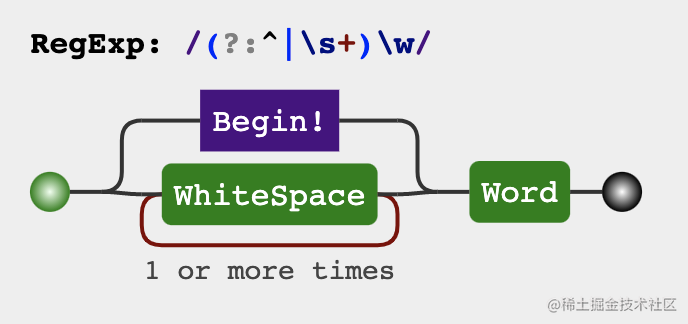### 分析过程

``````
const capitalize = (string) => {
const capitalizeRegex = /(?:^|\s+)\w/g

return string.toLowerCase().replace(capitalizeRegex, (match) => match.toUpperCase())
}

console.log(capitalize('hello world')) // Hello World
console.log(capitalize('hello WORLD')) // Hello World

## 5.# 通过name获取url query参数

### 正则结果

``````const getQueryByName = (name) => {
const queryNameRegex = new RegExp(`[?&]\${name}=([^&]*)(&|\$)`)
const queryNameMatch = window.location.search.match(queryNameRegex)
// 一般都会通过decodeURIComponent解码处理
return queryNameMatch ? decodeURIComponent(queryNameMatch) : ''
}

### 分析过程

url query上的参数 `name=前端胖头鱼` 所处的位置可能是

1. `紧跟着问号` ?name=前端胖头鱼&sex=boy

2. `在最后的位置` ?sex=boy&name=前端胖头鱼

3. `在1和2之间` ?sex=boy&name=前端胖头鱼&age=100

1. name前面只能是?或者&
2. value的值可以除了是&以为的任意东西
3. value后面只能是跟着&或者是结束位置
``````
const getQueryByName = (name) => {
const queryNameRegex = new RegExp(`[?&]\${name}=([^&]*)(?:&|\$)`)
const queryNameMatch = window.location.search.match(queryNameRegex)
// 一般都会通过decodeURIComponent解码处理
return queryNameMatch ? decodeURIComponent(queryNameMatch) : ''
}
// 1. name在最前面
// https://juejin.cn/?name=前端胖头鱼&sex=boy
console.log(getQueryByName('name')) // 前端胖头鱼

// 2. name在最后
// https://juejin.cn/?sex=boy&name=前端胖头鱼
console.log(getQueryByName('name')) // 前端胖头鱼

// 2. name在中间
// https://juejin.cn/?sex=boy&name=前端胖头鱼&age=100
console.log(getQueryByName('name')) // 前端胖头鱼

## 6.# 提取连续重复的字符

### 正则结果

``````const collectRepeatStr = (str) => {
let repeatStrs = []
const repeatRe = /(.+)\1+/g

str.replace(repeatRe, (\$0, \$1) => {
\$1 && repeatStrs.push(\$1)
})

return repeatStrs
}

### 分析过程

1. 连续重复的字符
2. 连续重复的字符数的长度是不限的（如23、45是两位、6是一位）

`那什么是连续重复呢？`

11是连续重复、22也是连续重复、111当然也是。也就是说某些字符X之后一定也是跟着X，就叫连续重复。如果很明确知道X是就是1，那么`/11+/`也就可以匹配了，但关键是这里的X是不明确的，怎么办呢？。

``````// 这里的X可用.来表示，即所有的字符，并用括号进行引用，紧跟着反向应用\1，也就是体现了连续重复的意思啦
let repeatRe = /(.)\1/

console.log(repeatRe.test('11')) // true
console.log(repeatRe.test('22')) // true
console.log(repeatRe.test('333')) // true
console.log(repeatRe.test('123')) // false

``````
let repeatRe = /(.+)\1+/

console.log(repeatRe.test('11')) // true
console.log(repeatRe.test('22')) // true
console.log(repeatRe.test('333')) // true
console.log(repeatRe.test('454545')) // true
console.log(repeatRe.test('124')) // false

``````
const collectRepeatStr = (str) => {
let repeatStrs = []
const repeatRe = /(.+)\1+/g
// 很多时候replace并不是用来做替换，而是做数据提取用
str.replace(repeatRe, (\$0, \$1) => {
\$1 && repeatStrs.push(\$1)
})

return repeatStrs
}

console.log(collectRepeatStr('11')) // ["1"]
console.log(collectRepeatStr('12323')) // ["23"]
console.log(collectRepeatStr('12323454545666')) // ["23", "45", "6"]

## 7.# 实现一个trim函数

### 正则结果

``````// 去除空格法
const trim = (str) => {
return str.replace(/^\s*|\s*\$/g, '')
}
// 提取非空格法
const trim = (str) => {
return str.replace(/^\s*(.*?)\s*\$/g, '\$1')
}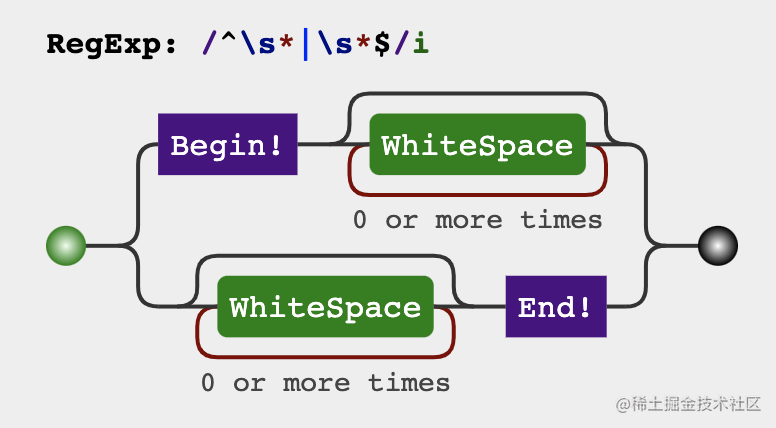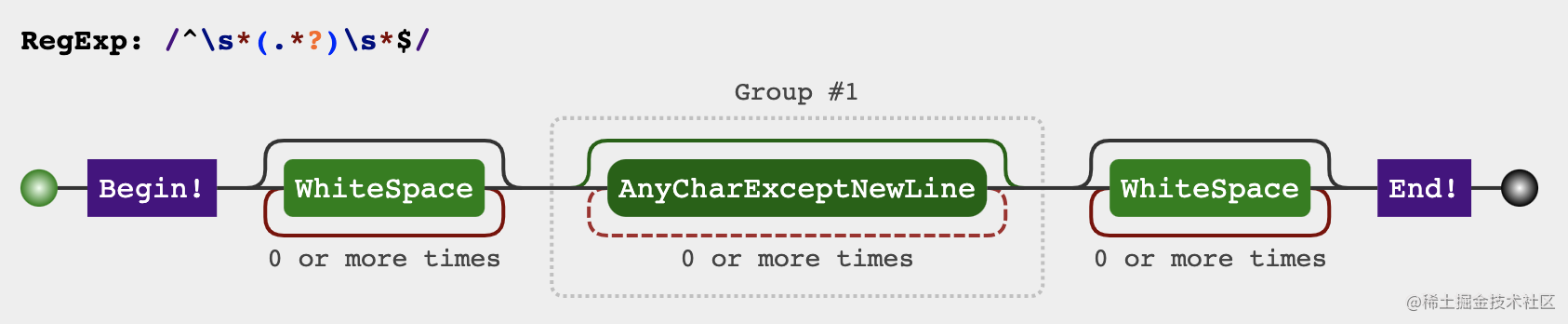### 分析过程

``````
const trim = (str) => {
return str.replace(/^\s*|\s*\$/g, '')
}

console.log(trim('  前端胖头鱼')) // 前端胖头鱼
console.log(trim('前端胖头鱼  ')) // 前端胖头鱼
console.log(trim('  前端胖头鱼  ')) // 前端胖头鱼
console.log(trim('  前端 胖头鱼  ')) // 前端 胖头鱼

``````
const trim = (str) => {
return str.replace(/^\s*(.*?)\s*\$/g, '\$1')
}

console.log(trim('  前端胖头鱼')) // 前端胖头鱼
console.log(trim('前端胖头鱼  ')) // 前端胖头鱼
console.log(trim('  前端胖头鱼  ')) // 前端胖头鱼
console.log(trim('  前端 胖头鱼  ')) // 前端 胖头鱼

## 结尾

• scq000
4年前
•• vortesnail
1年前
• 伊人a
1年前• jsliang
4年前
• 子弈
3年前
• VincentKo
4年前
• chroot
4年前
• CUGGZ
2年前•••